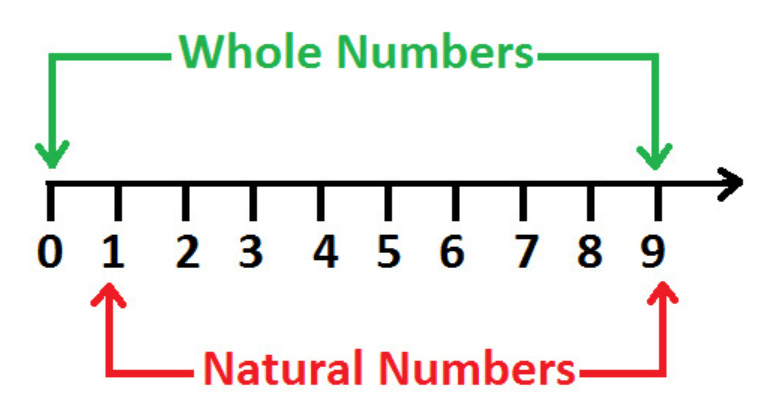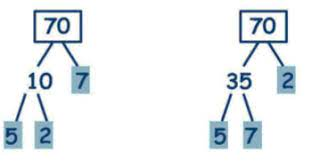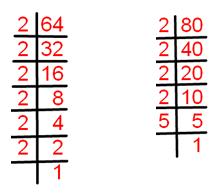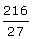Courses

# Chapter Notes - Playing with Numbers Class 6 Notes | EduRev

## Class 6 : Chapter Notes - Playing with Numbers Class 6 Notes | EduRev

The document Chapter Notes - Playing with Numbers Class 6 Notes | EduRev is a part of the Class 6 Course Mathematics (Maths) Class 6.
All you need of Class 6 at this link: Class 6

What is a Number?

A number is defined as an arithmetical value, expressed by a word, symbol, and figures. These numbers can be written in single digits, double digits, three digits in the generalized form.What are the Different Types of Numbers?

A number system is a system of writing for expressing numbers. According to the number system, the different types of a number includes:

• Prime numbers
• Even numbers
• Odd numbers
• Whole numbers
• Natural numbers
• Composite numbers

Lets us look into some solved example problems:
Q1. Write all the factors of 65
Ans: 65 is a composite number.
65 = 1 × 65
5 x 13 = 65
Factors of 65: 1, 5, 13, 65.

Q2. Find the common factors of 850 and 680
Ans: The common factors of 850 and 680 are 2, 5, and 17.

What are Factors, Perfect Numbers & Multiples?

Factors

A factor of a number is an exact divisor of that number.

Example: 1, 2, 3, and 6 are the factors of 6.

Properties of Factors

Properties of factors of a number:

• 1 is a factor of every number.
• Every number is a factor of itself.
• Every factor of a number is an exact divisor of that number.
• Every factor is less than or equal to the given number.
• The number of factors of a given number are finite.

Perfect numbers

A number for which the sum of all its factors is equal to twice the number is called a perfect number.

Example: Factors of 28 are 1, 2, 4, 7, 14 and 28.
Here, 1 + 2 + 4 + 7 + 14 + 28 = 56 = 2 × 28
Therefore, the sum of factors of 28 is equal to twice the number 28.

Multiples

Multiples of a number are those numbers that we get on multiplying the number by any integer.

Example: Multiples of 3 are 6, 9, 12, 15, 18, etc.

Properties of Multiples

Properties of multiples of a number:

• Every multiple of a number is greater than or equal to that number.
• The number of multiples of a given number is infinite.
• Every number is a multiple of itself.

Lets us look into some solved example problems of Factors and Multiples:

Q.1. List the first 5 multiples of 5.

Ans: First 5 multiples of 5 are : 10,15,20,25,30

Q.2. Which number is a factor of 10 among the numbers 3,4,5 & 8?

Ans: 5 is a factor of 10 among the numbers 3,4,5 & 8. If you can multiply two whole numbers to create a third number, those two numbers are factors of the third. 2 x 5 = 10, so 2 and 5 are factors of 10

Prime and Composite Numbers

Prime numbers

Numbers other than 1 whose only factors are 1 and the number itself are called Prime numbers.
Example: 2, 3, 5, 7 etc.

Composite numbers

Numbers having more than two factors are called Composite numbers.
Example: 4, 6, 8, etc.

Tests for Divisibility of Numbers

Divisibility Tests

A divisibility rule is a method of determining whether a given integer is divisible by a fixed divisor without performing division, usually by examining its digits.

We have divisibility rules for 2, 3, 4, 5, 6, 7, 8, 9, 10, and 11.

Divisibility tests for 2
If one’s digit of a number is 0,2,4,6 or 8, then the number is divisible by 2.
Example: 12, 34, 56, and 78.

Divisibility tests for 4
A number with 3 or more digits is divisible by 4 if the number formed by its last two digits (i.e. ones and tens) is divisible by 4.

Example: 1396 is divisible by 4 since its last two digits i.e. 36 is divisible by 4.

Divisibility tests for 3
A number is divisible by 3 if the sum of its digits is divisible by 3.
Example: Take 27.
Sum of its digits = 2+7= 9, which is divisible by 3.
Therefore, 27 is divisible by 3.

Divisibility tests for 5
If the one’s digit of a number is either 5 or 0, then it is divisible by 5.
Example: 75, 90, 100, and 125.

Divisibility tests for 8
A number with 4 or more digits is divisible by 8, if the number formed by its last three digits is divisible by 8.
Example: 73512 is divisible by 8 since its last three digits i.e. 512 is divisible by 8.

Try yourself:The number 5790176 is divisible by

Divisibility tests for 6
If a number is divisible by 2 and 3 both, then it is divisible by 6 also.
Example: 120 is divisible by 2 and 3. Therefore, it is divisible by 6 also.

Divisibility tests for 7
Double the last digit and subtract it from the remaining leading cut number. If the result is divisible by 7, then the original number is divisible by 7. Example: 826 is divisible by 7 since, 82 – (6 × 2) = 82 – 12 =70, which is divisible by 7.

Divisibility tests for 9
A number is divisible by 9 if the sum of its digits is divisible by 9.
Example: Consider 126.
Sum of its digits = 1+2+6 = 9, which is divisible by 9.
Therefore, 126 is divisible by 9.

Divisibility tests for 11
Find difference between sum of digits at odd places (from the right) and sum of digits at even places (from the right) of a number. If the difference is either 0 or divisible by 11, then the number is divisible by 11.
Example: 1234321 is divisible by 11 since, (1+3+3+1) – (2+4+2) = 8 – 8 = 0, which is divisible by 11.

Divisibility tests for 10
If one’s digit of a number is 0, then the number is divisible by 10.
Example: 10, 20, 30, and 40.

Common Factors and Common Multiples

Common Factors

The factors of 4 are 1, 2, and 4.
The factors of 18 are 1, 2, 3, 6, 9, and 18.
The numbers 1 and 2 are common factors of both 4 and 18.

Common Multiples

Multiples of 3 are 3, 6, 9, 12, 15, 18,….
Multiples of 5 are 5, 10, 15, 20, 25, 30,…
Multiples of 6 are 6, 12, 18, 24, 30, 36,…
Therefore, common multiples of 3, 5, and 6 are 30, 60,….

Prime Factorization

When a number is expressed as a product of prime numbers, factorization is called prime factorization.
Example: Prime factorization of 36 is 2×2×3×3.

There are two methods to find the prime factors of a number-

1. Prime factorization using a factor tree

We can find the prime factors of 70 in two ways.The prime factors of 70 are 2, 5 and 7 in both cases.

2. Repeated Division Method

Find the prime factorization of 64 and 80.The prime factorization of 64 is 2 × 2 × 2 × 2 × 2 × 2.

The prime factorization of 80 is 2 × 2 × 2 × 2 × 5.

Highest Common Factor (HCF)

The Highest Common Factor (HCF) of two or more given numbers is the highest (or greatest) of their common factors. It is also known as the Greatest Common Divisor (GCD).

Example: Consider two numbers 12 and 20. Factors of 12 are 1, 2, 3, 4, 6, and 12. Factors of 20 are 1, 2, 4, 5, 10, and 20. The common factors of 12 and 20 are 1, 2, and 4. The highest of these is 4. So, 4 is the HGF of 12 and 20.

Try yourself:The HCF of two numbers is 28 and their LCM is 336. If one number is 112, then the other number is ________.

Lowest Common Multiple (LCM)

The Lowest Common Multiple (LCM) of two or more given numbers is the lowest (or smallest or least) of their common multiples.

Example: Consider two numbers 12 and 20.

Multiples of 12 are 12, 24, 36, 48, 60, 72, 84, 96, 108, 120, …

Multiples of 20 are 20, 40, 60, 80, 100, 120, ……..

The common multiples of 12 and 20 are 60, 120,…

The lowest of these is 60.

So, 60 is the LCM of 12 and 20.

Solved Questions for You

Q.1. The sum of the two numbers is 216 and HCF is 27. Find the numbers.
Ans: Let the numbers are 27a and 27b.
27a + 27 b = 216
a + b == 8
Co-primes with sum 8 are (1,7) and (3,5)
Required numbers are (27 x 1 ), (27 x 7) or (27 x 3 ), (27 x 5)
i.e. (27,189) or (81 x 135)

Q.2. Use the divisibility test to determine whether the number 1258 is divisible by 6.

Ans:  Given number is 1258.
Its units digit is 8, which is divisible by 2. So, 1258 is divisible by 2.
Sum of its digits = 1 + 2 + 5 + 8 = 16, which is not divisible by 3. So, 1258 is not divisible by 3.
Since 1258 is divisible by 2 but not by 3, it is not divisible by 6.

Q.3. Find the prime factorization of 2520.

Ans: 2520 = 2 × 1260
= 2 × 2 × 630
= 2 × 2 × 2 × 315
= 2 × 2 × 2 × 3 × 105
= 2 × 2 × 2 × 3 × 3 × 35
= 2 × 2 × 2 × 3 × 3 × 5 × 7

Offer running on EduRev: Apply code STAYHOME200 to get INR 200 off on our premium plan EduRev Infinity!

## Mathematics (Maths) Class 6

185 videos|229 docs|43 tests

,

,

,

,

,

,

,

,

,

,

,

,

,

,

,

,

,

,

,

,

,

;